## 1 The Discrete Kalman Filter

In 1960, R.E. Kalman published his famous paper describing a recursive solution to the discrete-data linear filtering problem [Kalman60]. Since that time, due in large part to advances in digital computing, the Kalman filter has been the subject of extensive research and application, particularly in the area of autonomous or assisted navigation. A very "friendly" introduction to the general idea of the Kalman filter can be found in Chapter 1 of [Maybeck79], while a more complete introductory discussion can be found in [Sorenson70], which also contains some interesting historical narrative. More extensive references include [Gelb74], [Maybeck79], [Lewis86], [Brown92], and [Jacobs93].

#### The Process to be Estimated

The Kalman filter addresses the general problem of trying to estimate the state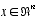of a discrete-time controlled process that is governed by the linear stochastic difference equation

#####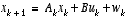, (1.1)

with a measurement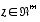that is

#####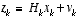. (1.2)

The random variables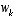and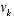represent the process and measurement noise (respectively). They are assumed to be independent (of each other), white, and with normal probability distributions

#####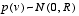. (1.4)

The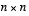matrix A in the difference equation (1.1) relates the state at time step k to the state at step k+1, in the absence of either a driving function or process noise. The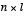matrix B relates the control input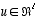to the state x. The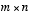matrix H in the measurement equation (1.2) relates the state to the measurement zk.

#### The Computational Origins of the Filter

We define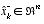(note the "super minus") to be our a priori state estimate at step k given knowledge of the process prior to step k, and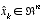to be our a posteriori state estimate at step k given measurement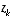. We can then define a priori and a posteriori estimate errors as

#####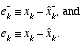The a priori estimate error covariance is then

#####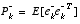, (1.5)

and the a posteriori estimate error covariance is

#####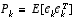. (1.6)

In deriving the equations for the Kalman filter, we begin with the goal of finding an equation that computes an a posteriori state estimate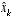as a linear combination of an a priori estimate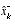and a weighted difference between an actual measurementand a measurement prediction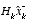as shown below in (1.7) . Some justification for (1.7) is given in "The Probabilistic Origins of the Filter" found below.

#####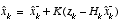(1.7)

The difference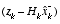in (1.7) is called the measurement innovation, or the residual. The residual reflects the discrepancy between the predicted measurementand the actual measurement. A residual of zero means that the two are in complete agreement.

The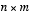matrix K in (1.7) is chosen to be the gain or blending factor that minimizes the a posteriori error covariance (1.6) . This minimization can be accomplished by first substituting (1.7) into the above definition for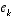, substituting that into (1.6) , performing the indicated expectations, taking the derivative of the trace of the result with respect to K, setting that result equal to zero, and then solving for K. For more details see [Maybeck79], [Brown92], or [Jacobs93]. One form of the resulting K that minimizes (1.6) is given by 1

#####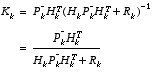. (1.8)

Looking at (1.8) we see that as the measurement error covariance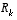approaches zero, the gain K weights the residual more heavily. Specifically,

#####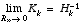.

On the other hand, as the a priori estimate error covariance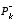approaches zero, the gain K weights the residual less heavily. Specifically,

#####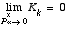.

Another way of thinking about the weighting by K is that as the measurement error covarianceapproaches zero, the actual measurementis "trusted" more and more, while the predicted measurementis trusted less and less. On the other hand, as the a priori estimate error covarianceapproaches zero the actual measurementis trusted less and less, while the predicted measurementis trusted more and more.

#### The Probabilistic Origins of the Filter

The justification for (1.7) is rooted in the probability of the a priori estimateconditioned on all prior measurements(Bayes' rule). For now let it suffice to point out that the Kalman filter maintains the first two moments of the state distribution,

#####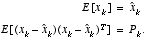The a posteriori state estimate (1.7) reflects the mean (the first moment) of the state distribution-- it is normally distributed if the conditions of (1.3) and (1.4) are met. The a posteriori estimate error covariance (1.6) reflects the variance of the state distribution (the second non-central moment). In other words,

#####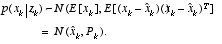.

For more details on the probabilistic origins of the Kalman filter, see [Maybeck79], [Brown92], or [Jacobs93].

#### The Discrete Kalman Filter Algorithm

We will begin this section with a broad overview, covering the "high-level" operation of one form of the discrete Kalman filter (see the previous footnote). After presenting this high-level view, we will narrow the focus to the specific equations and their use in this version of the filter.

The Kalman filter estimates a process by using a form of feedback control: the filter estimates the process state at some time and then obtains feedback in the form of (noisy) measurements. As such, the equations for the Kalman filter fall into two groups: time update equations and measurement update equations. The time update equations are responsible for projecting forward (in time) the current state and error covariance estimates to obtain the a priori estimates for the next time step. The measurement update equations are responsible for the feedback--i.e. for incorporating a new measurement into the a priori estimate to obtain an improved a posteriori estimate.

The time update equations can also be thought of as predictor equations, while the measurement update equations can be thought of as corrector equations. Indeed the final estimation algorithm resembles that of a predictor-corrector algorithm for solving numerical problems as shown below in Figure 1-1 .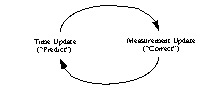```
Figure 1-1.
The ongoing discrete Kalman filter cycle. The time update projects the current state estimate ahead in time. The measurement update adjusts the projected estimate by an actual measurement at that time.```

The specific equations for the time and measurement updates are presented below in Table 1-1 and Table 1-2 .

######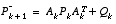(1.10)

Again notice how the time update equations in Table 1-1 project the state and covariance estimates from time step k to step k+1.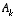and B are from (1.1) , while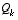is from (1.3) . Initial conditions for the filter are discussed in the earlier references.

######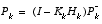(1.13)

The first task during the measurement update is to compute the Kalman gain,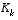. Notice that the equation given here as (1.11) is the same as (1.8) . The next step is to actually measure the process to obtain, and then to generate an a posteriori state estimate by incorporating the measurement as in (1.12) . Again (1.12) is simply (1.7) repeated here for completeness. The final step is to obtain an a posteriori error covariance estimate via (1.13) .

After each time and measurement update pair, the process is repeated with the previous a posteriori estimates used to project or predict the new a priori estimates. This recursive nature is one of the very appealing features of the Kalman filter--it makes practical implementations much more feasible than (for example) an implementation of a Weiner filter [Brown92] which is designed to operate on all of the data directly for each estimate. The Kalman filter instead recursively conditions the current estimate on all of the past measurements. Figure 1-2 below offers a complete picture of the operation of the filter, combining the high-level diagram of Figure 1-1 with the equations from Table 1-1 and Table 1-2 .

#### Filter Parameters and Tuning

In the actual implementation of the filter, each of the measurement error covariance matrixand the process noise(given by (1.4) and (1.3) respectively) might be measured prior to operation of the filter. In the case of the measurement error covariancein particular this makes sense--because we need to be able to measure the process (while operating the filter) we should generally be able to take some off-line sample measurements in order to determine the variance of the measurement error.

In the case of, often times the choice is less deterministic. For example, this noise source is often used to represent the uncertainty in the process model (1.1) . Sometimes a very poor model can be used simply by "injecting" enough uncertainty via the selection of. Certainly in this case one would hope that the measurements of the process would be reliable.

In either case, whether or not we have a rational basis for choosing the parameters, often times superior filter performance (statistically speaking) can be obtained by "tuning" the filter parametersand. The tuning is usually performed off-line, frequently with the help of another (distinct) Kalman filter.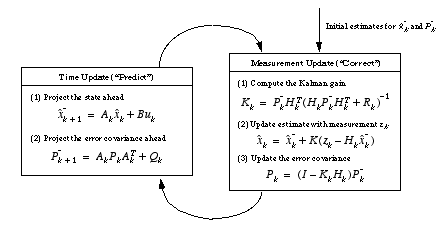```
Figure 1-2.
A complete picture of the operation of the Kalman filter, combining the high-level diagram of Figure 1-1
with the equations from Table 1-1
and Table 1-2
.```

In closing we note that under conditions whereand.are constant, both the estimation error covariance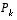and the Kalman gainwill stabilize quickly and then remain constant (see the filter update equations in Figure 1-2 ). If this is the case, these parameters can be pre-computed by either running the filter off-line, or for example by solving (1.10) for the steady-state value ofby definingand solving for.

It is frequently the case however that the measurement error (in particular) does not remain constant. For example, when sighting beacons in our optoelectronic tracker ceiling panels, the noise in measurements of nearby beacons will be smaller than that in far-away beacons. Also, the process noiseis sometimes changed dynamically during filter operation in order to adjust to different dynamics. For example, in the case of tracking the head of a user of a 3D virtual environment we might reduce the magnitude ofif the user seems to be moving slowly, and increase the magnitude if the dynamics start changing rapidly. In such a casecan be used to model not only the uncertainty in the model, but also the uncertainty of the user's intentions.